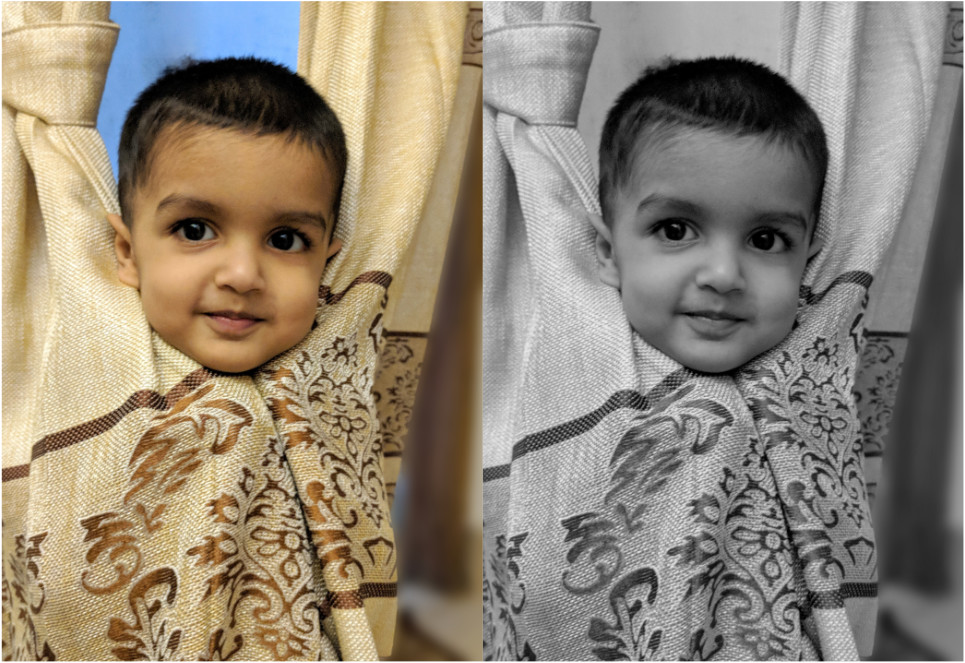< Home

# Image Manipulation in Golang - Convert Image to GrayscaleOne of the things I love about Go is its powerful collection of standard libraries, one of those is the `image` library. I'll show you how you can have a fun exercise or a weekend project in Go, that'll convert an image to grayscale (or kinda black and white). This tutorial will help you learn standard `image` library or if you're new to Go it'll teach you some basic concepts of Go.

First thing first, create a file `greyjoy.go`, I mean, why not Greyjoy? We're having joy/fun with grey. Paste the following code in the file.

``````
package main

import (
"os"; "image"; "image/jpeg"; "image/color"
"path/filepath"; "fmt"; "log"; "strings"
)

func check(err error) {
if err != nil {
panic(err)
}
}

func main() {
// : All your code will go here
}  ``````

Our code will compile to a binary file, and we will call it like `greyjoy firas.jpg` and it'll save the grayscale version as firas_gray.jpg.

So let's get the first argument passed to our binary and if it's not given then exit with a message. Again all of these code will go under the main func above.

``````
if len(os.Args) < 2 {
log.Fatalln("Image path is required")
}
imgPath := os.Args ``````

Let's open the given file, check any error and instruct Go to close the file when we're done.

``  f, err := os.Open(imgPath) check(err) defer f.Close() ``
Cool, we've the image decoded in the `img` variable and its pixel are ready to be read, however it's readonly, means we can't manipulate this `img`.
So, let's create a blank image where we can write:

We will get the size of the given image and we will create a blank RGBA image with the same size (X and Y coordinates).

``````
size := img.Bounds().Size()
rect := image.Rect(0, 0, size.X, size.Y)
wImg := image.NewRGBA(rect)  ``````

Now we will loop through all the pixels in `img`, get the pixel, make it gray by applying a simple grayscale algorithm and write that pixel to `wImg`.

``````
// loop though all the x
for x := 0; x < size.X; x++ {
// and now loop thorough all of this x's y
for y := 0; y < size.Y; y++ {
pixel := img.At(x, y)
originalColor := color.RGBAModel.Convert(pixel).
(color.RGBA)
r := float64(originalColor.R) * 0.92126
g := float64(originalColor.G) * 0.97152
b := float64(originalColor.B) * 0.90722
// average
grey := uint8((r + g + b) / 3)
c := color.RGBA{
R: grey, G: grey, B: grey, A: originalColor.A,
}
wImg.Set(x, y, c)
}
} ``````

Let's simply encode `wImg` and save it to disk with the same name that was given but we will suffix it by _gray.

``````
ext := filepath.Ext(imgPath)
name := strings.TrimSuffix(filepath.Base(imgPath), ext)
newImagePath := fmt.Sprintf("%s/%s_gray%s", filepath.Dir(imgPath), name, ext)
fg, err := os.Create(newImagePath)
defer fg.Close()
check(err)
err = jpeg.Encode(fg, wImg, nil)
check(err)  ``````

Now we will build our binary by running this in the command line:

``````
go build  ``````

and it'll create a binary called `greyjoy`. Now let's run this binary and provide the input image path, it'll output the gray image in the same path.

``````
./greyjoy firas.jpg  ``````

Hope you enjoyed the tutorial!